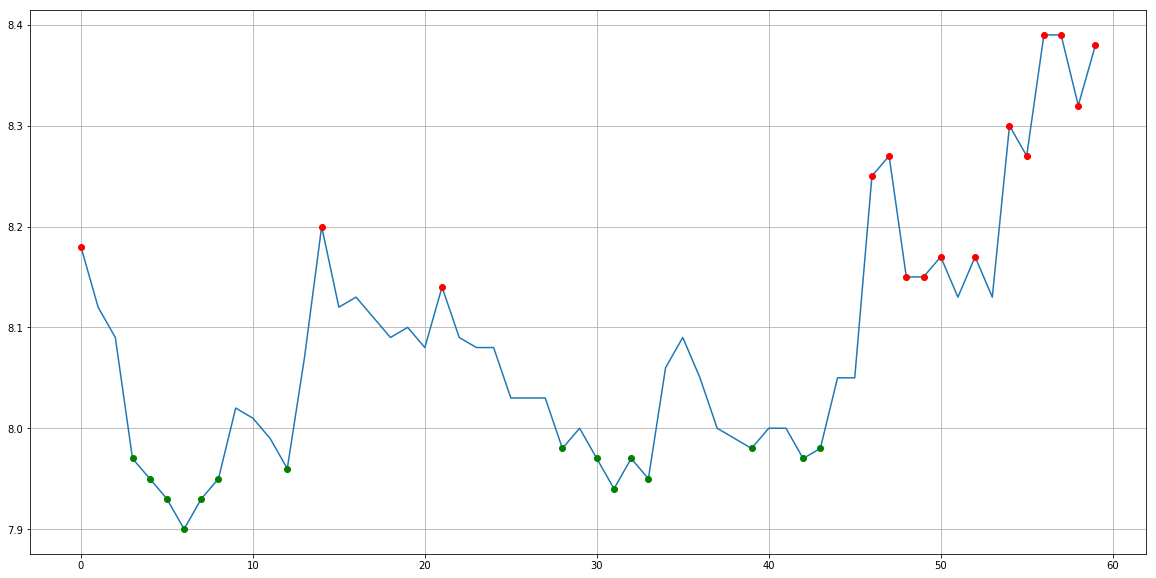# pandas

pandas is an open source, BSD-licensed library providing high-performance, easy-to-use data structures and data analysis tools for the Python programming language.

pandas is a NumFOCUS sponsored project.

## 功能简介

pandas是python环境下最有名的数据统计包，而DataFrame翻译为数据框，是一种数据组织方式。

## 和 Numpy 的区别

2. NumPy简介：N维数组容器NumPy系统是Python的一种开源的数值计算扩展。这种工具可用来存储和处理大型矩阵，比Python自身的嵌套列表（nested list structure)结构要高效的多（该结构也可以用来表示矩阵（matrix））。据说NumPy将Python相当于变成一种免费的更强大的MatLab系统。

3. Pandas简介：表格容器 pandas 是基于NumPy 的一种工具，该工具是为了解决数据分析任务而创建的。Pandas 纳入了大量库和一些标准的数据模型，提供了高效地操作大型数据集所需的工具。pandas提供了大量快速便捷地处理数据的函数和方法。使Python成为强大而高效的数据分析环境的重要因素之一。

# 快速开始

## install

pip3 install pandas


## import

import numpy as np
import pandas as pd


## 创建一个序列

>>> s = pd.Series([1, 3, 5, np.nan, 6, 8])
>>> s
0    1.0
1    3.0
2    5.0
3    NaN
4    6.0
5    8.0
dtype: float64


# 实战例子

## 简单的股票分析

python 真的是一个大宝库，里面的api真的超级多，而且使用超级方便。

## 代码

import numpy as np
import pandas as pd
import matplotlib.pyplot as plt
import heapq
import tushare as ts
import datetime

#使用平安银行的数据
date_end = datetime.datetime(int(2017), int(11), int(6))
date_start = (date_end + datetime.timedelta(days=-90)).strftime("%Y-%m-%d") #往前90 天数据
date_end = date_end.strftime("%Y-%m-%d")
code = "601857"
#测试
print(code, date_start, date_end)

#假设股票数据
# open, high, close, low, volume, price_change, p_change, ma5, ma10, ma20, v_ma5, v_ma10, v_ma20, turnover
stock = ts.get_hist_data(code, start=date_start, end=date_end)
stock = stock.sort_index(0)  # 将数据按照日期排序下。

#打印头和尾部数据
print(len(stock))
print(stock.tail(1))

def wave_guess(arr):
wn = int(len(arr)/4) #没有经验数据，先设置成1/4。
print(wn)
#计算最小的N个值，也就是认为是波谷
wave_crest = heapq.nlargest(wn, enumerate(arr), key=lambda x: x)
wave_crest_mean = pd.DataFrame(wave_crest).mean()

#计算最大的5个值，也认为是波峰
wave_base = heapq.nsmallest(wn, enumerate(arr), key=lambda x: x)
wave_base_mean = pd.DataFrame(wave_base).mean()

print("######### result #########")
#波峰，波谷的平均值的差，是波动周期，对于股票就是天。
wave_period = abs(int( wave_crest_mean - wave_base_mean))
print("wave_period_day:", wave_period)
print("wave_crest_mean:", round(wave_crest_mean,2))
print("wave_base_mean:", round(wave_base_mean,2))

############### 以下为画图显示用 ###############
wave_crest_x = [] #波峰x
wave_crest_y = [] #波峰y
for i,j in wave_crest:
wave_crest_x.append(i)
wave_crest_y.append(j)

wave_base_x = [] #波谷x
wave_base_y = [] #波谷y
for i,j in wave_base:
wave_base_x.append(i)
wave_base_y.append(j)

#将原始数据和波峰，波谷画到一张图上
plt.figure(figsize=(20,10))
plt.plot(arr)
plt.plot(wave_base_x, wave_base_y, 'go')#红色的点
plt.plot(wave_crest_x, wave_crest_y, 'ro')#蓝色的点
plt.grid()
plt.show()

#使用收盘价格画图：
arr1 = pd.Series(stock["close"].values)
wave_guess(arr1)

arr2 = pd.Series(stock["ma5"].values)
wave_guess(arr2)

arr3 = pd.Series(stock["v_ma5"].values)
wave_guess(arr3)


## 效果图# 个人感受

pandas 是非常强大的量化计算工具，适合深入学习。